# 1. Objective

You will learn how to interface the real world with MCUs, details about the difference between digital input and analogue input and how to read input from a potentiometer.

# 2. Interfacing with real world

Monitoring and controlling the real environment is commonly analogue in nature. To interface with the environment Analogue to Digital Conversion (ADC) and Digital to Analogue Conversion (DAC) are required.In analogue to digital signal, the error is mainly due to sampling and quantisation.

3. Potentiometer

Problem: Write a program to get the reading of the potentiometer.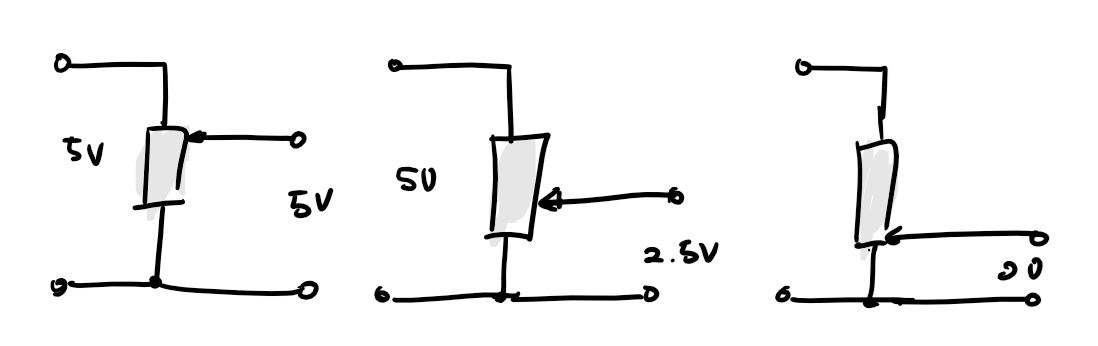The output of a potentiometer that we are using is between 0 and 5 volt and it is manually adjustable. In this case, we do not have any choice but to use ADC to read the potentiometer output.

Let's write the programm.

First of all, initize all the inputs and outputs.

To read the value we will need 8 or 16 bit value, uint8_t poti_getValue8bit(void); or use double in Arduino IDE.

``` // Input pin int button =0; // select the pin for the button // Output pin int ledPin1 = 11; // select the pin for the LED1 int ledPin2 = 12; // select the pin for the LED2 int ledPin3 = 13; // select the pin for the LED3 // Input Potentiometer int sensorPin= A0; // select the pin for the potentiometer double voltageValue; void setup() {  // put your setup code here, to run once:  pinMode(ledPin1, OUTPUT); // declare the ledPin as an OUTPUT  pinMode(ledPin2, OUTPUT); // declare the ledPin as an OUTPUT  pinMode(ledPin3, OUTPUT); // declare the ledPin as an OUTPUT  pinMode(button, INPUT); // declare the button as an INPUT  // initialize serial communication at 9600 bits per second:  Serial.begin(9600); } void loop() {  // read the input on analog pin 1:  int sensorValue = analogRead(sensorPin);  voltageValue = ((double)sensorValue)/1023*5;  // print out the value you read:  //Serial.print("SensorValue=");  //Serial.println (sensorValue);  Serial.print("voltageValue=");  Serial.println (voltageValue);  delay(100);  if ((voltageValue<1.66))//   digitalWrite(ledPin1, HIGH); // turn LED ON  else      digitalWrite(ledPin1, LOW); // turn LED OFF     if((voltageValue>1.66)&&(voltageValue<3.32))//   digitalWrite(ledPin2, HIGH); // turn LED ON  else      digitalWrite(ledPin2, LOW); // turn LED OFF     if ((voltageValue>3.32)&&(voltageValue<=5))   digitalWrite(ledPin3, HIGH); // turn LED ON  else     digitalWrite(ledPin3, LOW); // turn LED OFF } ```

To run this code I used a Satshakit-128 board.

Regarding the input of the potentiometer turn on the led as the potentiometer is turned the led is leveled up.

4. UltrasonicUltrasonic to measure distance with an led bars to indicate how long does it measures.

Operation Principles

Piezoelectric devices are commonly used in ultrasonic sensors which emit high frequency (above 20kHz) sound pulses at regular intervals. The reflection from nearby object is obtained by a receiver and then based on the speed of sound the flight time can be calculated.

Physics : active sensor, works on time of flight

Advantages : Range between 12 cm up to 5 m, inexpensive (4Euro), small

Disadvantages : Specular reflection, crosstalk, foreshortening, high power consumption

Connect the triger pin and echo pin! The pulse a sound wave and wait until the sound is recieved, the interval time will be calculated to measure the distance.

# 5. PCB boardThis is the pinout from the datasheet of

ATmega 328P

.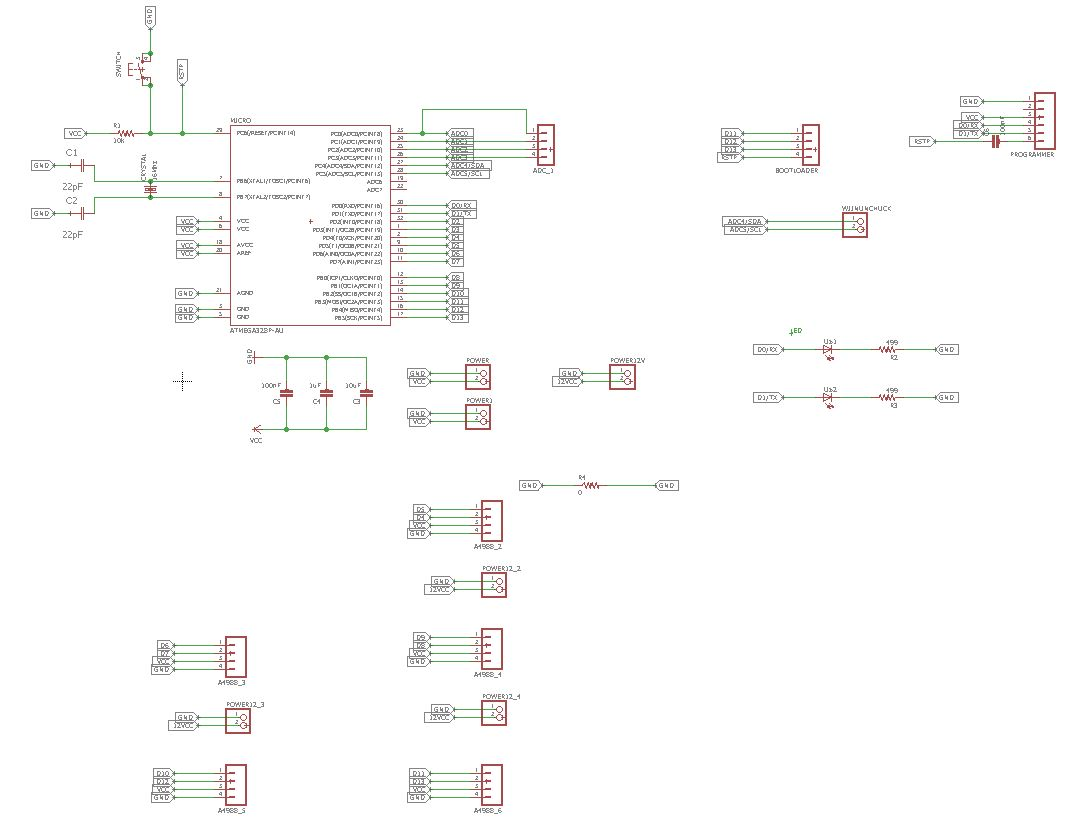This is the main board for my final project that can be connected easily to a FTDI and 5 stepper motor driver connections.

The modifications are adding FTDI connections, pins for bootloading the microcontroller and 5 stepper motor driver connections

• ♦FTDI connections are GND, nothing, VCC, RX pin, TX pin and reset to microcontroller.
• ♦Bootloader pins are GND,VCC,D13 and D12 to the microcontroller.
• ♦Stepper motor driver connections are connected to a digital pin for step, one digital pin for direction, VCC and GND.
•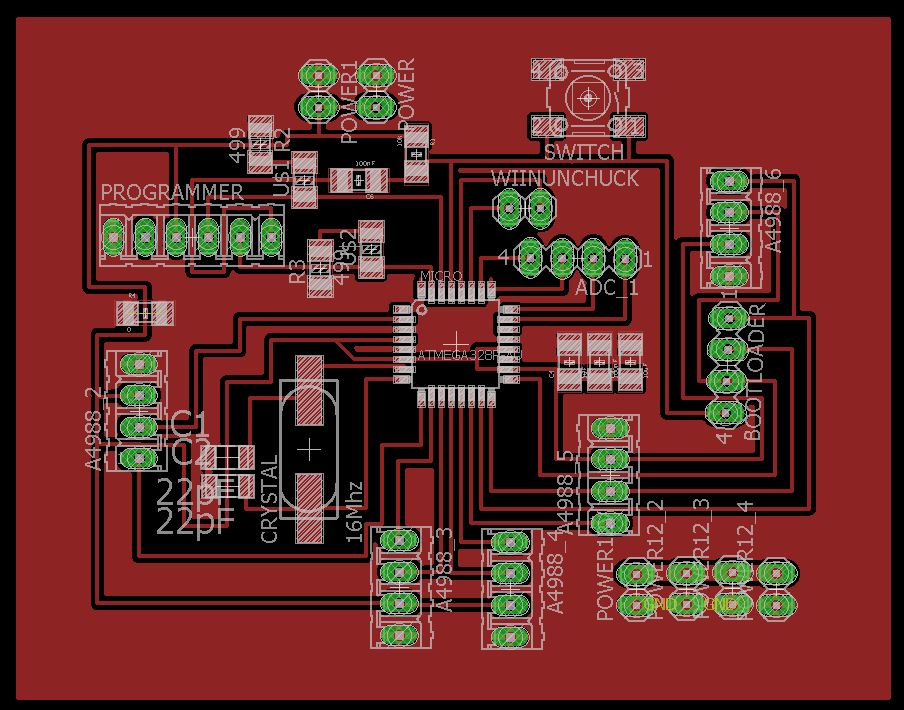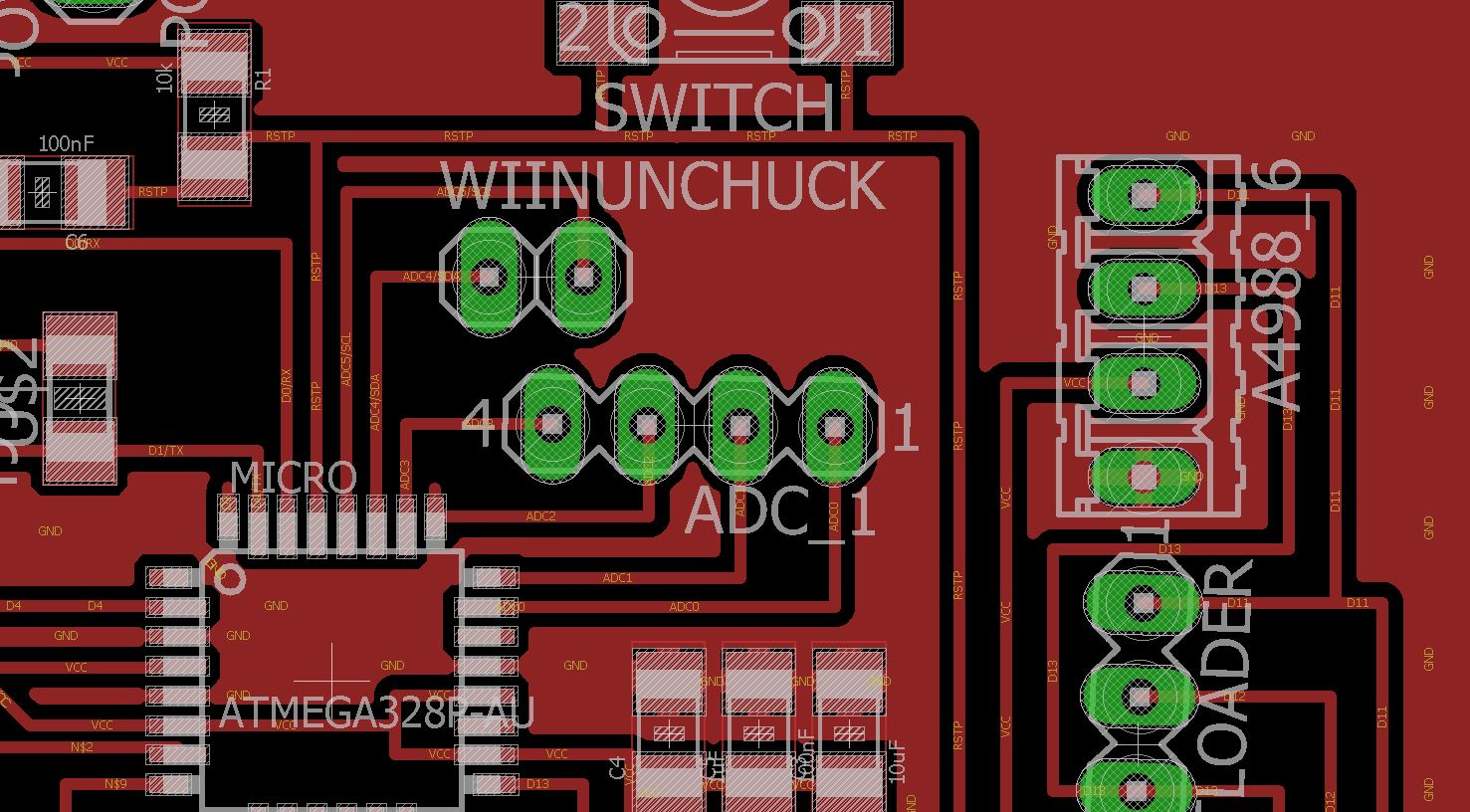This is the board!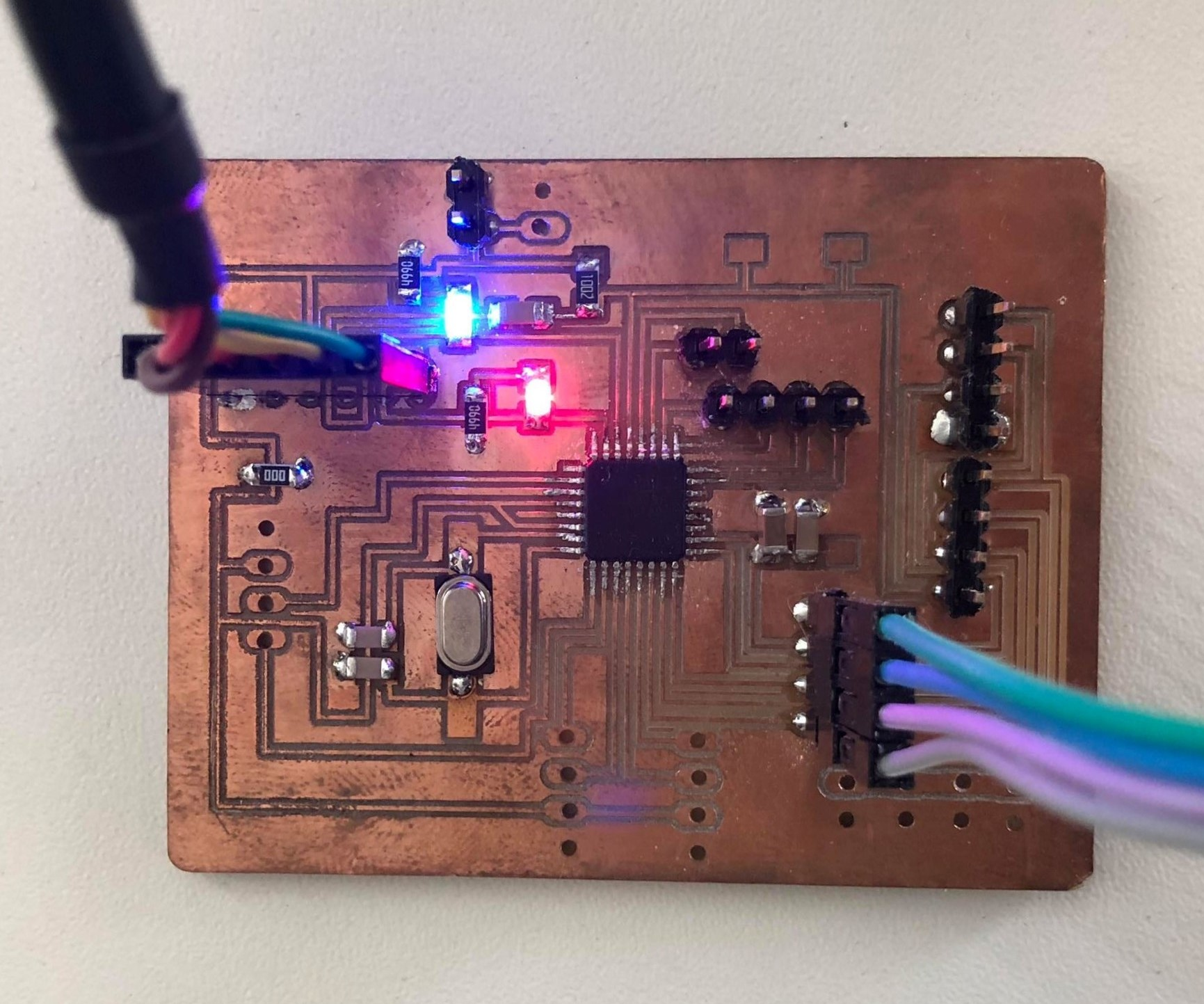# 6. Joystick

This week, I was trying to program a joystick. First of all, I was trying to get the input on the potentiometers in the joystick in x and y direction and by that I used a state machine to idetinfy where the user switch the joystick.

I thought that the original SatshaKit will fit nicely. It will create a mess of cable, therefore from the satshakit I modified the pins location so it can be connected directly to the ISP and easily connect to the joystick and a stepper motor driver for next week assignment.

Code :

``` // input pins const byte Xin = A0; // X input connected to A0 const byte Yin = A1; // Y input connected to A1 const byte Buttonin = 11; // pushbuttom input connected to Digital pin 7 // output pins (only if necessary) int ledPinBlue = 0; // select the pin for the blue LED PB0 int ledPinRed = 1; // select the pin for the red LED PB1 // state machine int state = 0; //threshold because of physical limitation 533 centre of joystick at x axis const int THRESHOLD_LOW_X = 523; const int THRESHOLD_HIGH_X = 543; //threshold because of physical limitation 522 centre of joystick at y axis const int THRESHOLD_LOW_Y = 512; const int THRESHOLD_HIGH_Y = 532; // joystick value int sensorValuex,sensorValuey, buttonState; void setup() {  // put your setup code here, to run once:  Serial.begin(9600); // initialize serial communication  //Input  pinMode (Buttonin, INPUT_PULLUP); // internal pull up resistor  pinMode (Xin, INPUT); // initialize Xin as INPUT  pinMode (Yin, INPUT); // initialize YZin as INPUT  //Output  pinMode(ledPinBlue, OUTPUT); // declare the ledPin as an OUTPUT  pinMode(ledPinRed, OUTPUT); // declare the ledPin as an OUTPUT } void loop() {  // put your main code here, to run repeatedly:  //readPotentiometer();  moveAxis(); } void readPotentiometer(){  sensorValuex = analogRead(Xin);  sensorValuey = analogRead(Yin);  buttonState = digitalRead(Buttonin);  /*  Serial.print(" Axis XY");  Serial.print(" X:");  Serial.print(sensorValuex);  Serial.print(" Y:");  Serial.println(sensorValuey);  */ } void moveAxis(){  if(state == 0){   readPotentiometer();   if(sensorValuex > THRESHOLD_HIGH_X){ //move right    state = 1;   }else if(sensorValuex < THRESHOLD_LOW_X){ //move left    state = 2;   }else if(sensorValuey > THRESHOLD_HIGH_Y){ //move down    state = 3;   }else if(sensorValuey < THRESHOLD_LOW_Y){ //move up    state = 4;   }  }else if(state ==1){   Serial.println("MOVE RIGHT");   readPotentiometer();   if((sensorValuex < THRESHOLD_HIGH_X) & & (sensorValuex > THRESHOLD_LOW_X)){    state = 0; // joy stick at the centre back to state 0  }  }else if(state ==2){   Serial.println("MOVE LEFT");   readPotentiometer();   if((sensorValuex < THRESHOLD_HIGH_X) & & (sensorValuex > THRESHOLD_LOW_X)){    state = 0; // joy stick at the centre back to state 0   }  }else if(state ==3){   Serial.println("MOVE DOWN");   readPotentiometer();   if((sensorValuey < THRESHOLD_HIGH_Y) & & (sensorValuey > THRESHOLD_LOW_Y)){    state = 0; // joy stick at the centre back to state 0   }  }else if(state ==4){   Serial.println("MOVE UP");   readPotentiometer();   if((sensorValuey < THRESHOLD_HIGH_Y) & & (sensorValuey > THRESHOLD_LOW_Y)){    state = 0; // joy stick at the centre back to state 0   }  } } ```

In the code above first of all the programm define all the pins that are connected to the microcontroller and then set up the inputs. in the function moveAxis it is where the state machine for the joystick programmed.

Video: